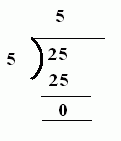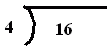Name: ___________________Date:___________________

 Email us to get an instant 20% discount on highly effective K-12 Math & English kwizNET Programs!

### Grade 4 - Mathematics5.4 Division using '')''

 Division can be written horizontally as 25/5 or 25 ÷ 5 Division problem may be written vertically as 5)25(Method: Divide the dividend by the divisor. The result is the quotient. Answer: 5 Directions: Divide the following. Also write at least 10 examples of your own.
 Q 1: Solve the following division problem.243484 Q 2: Solve the following division problem.8679 Q 3: Solve the following division problem.5689 Q 4: Solve the following division problem.7986 Q 5: Solve the following division problem.19131012 Q 6: Solve the following division problem.8346 Q 7: Solve the following division problem.909310 Q 8: Solve the following division problem.6978 Question 9: This question is available to subscribers only! Question 10: This question is available to subscribers only!